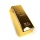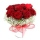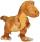# Prime numbers - 7th grade (12y) - math problems

1. QuotientFind quotient before the bracket - the largest divisor 51 a + 34 b + 68 121y-99z-33
2. NumberWhat number should be placed instead of the asterisk in number 702*8 to get a number divisible by 6?
3. The KingThe King wants to divide his sons equally. He has 42 rubies and 45 diamonds. How many sons and how will they share them?
4. Three linesAt 6 am, three bus lines are departing from the station. The first line has an interval of 24 minutes. The second line has an interval of 15 minutes. The third line runs at regular intervals of more than 1 minute. The third line runs at the same time as th
5. Prime factorsWrite 98 as product of prime factors
6. PexesoDrawing paper has dimensions of 60cm and 840mm. Pupils have to divide it into squares so that they can make a pexeso. What dimension must squares have if their side is to be larger than 3cm and less than 10cm?
7. Glass panelA rectangular glass panel with dimensions of 72 cm and 96 cm will cut the glazier on the largest square possible. What is the length of the side of each square? How many squares does the glazier cut?
8. Common divisorsFind all common divisors of numbers 30 and 45.
9. Wide fieldThe field is 203 meters wide 319 meter long, what is the greater length of the rope by which length and width can be measured and find the exact number of time.
10. LCD 2The least common denominator of 2/5, 1/2, and 3/4
11. BicyclesYou're the owner of the transport 's learning playground. Buy bicycles of two colors but you've got to spend accurately 120000 Kč. Blue bike costs 3600Kč and red bicycle 3200Kč.
12. 7 digit numberIf 3c54d10 is divisible by 330, what is the sum of c and d?
13. Dance groupThe dance group formed groups of 4, 5, and 6 members. Always one dancer remains. How many dancers were there in the whole group?
14. RectanglesHow many different rectangles can be made from 60 square tiles of 1 m square? Find the dimensions of these rectangles.
15. SchoolLess than 500 pupils attend school. When it is sorted into pairs, one pupil remains. Similarly, when sorted into 3, 4, 5 and 6 members team one remains. Sorted to seven members teams, no left behind. How many pupils are attending this school?
16. Three friendsThree friends had balls in ratio 2: 7: 4 at the start of the game. Could they have the same number of balls at the end of the game? Write 0, if not, or write the minimum number of balls they had together.
17. Two rectanglesI cut out two rectangles with 54 cm², 90 cm². Their sides are expressed in whole centimeters. If I put these rectangles together I get a rectangle with an area of 144 cm2. What dimensions can this large rectangle have? Write all options. Explain your calcu
18. The floristThe florist had 200 roses in the morning. During the day, more than half sold it. From the remaining roses, it will tie the bouquet. If a bouquet of 3, 4, 5 or 6 roses are bound, one always remains. How many roses from the morning shipment sold?
19. DinosaursMore than 30 and less than 60 dinosaurs have met at the pond. A quarter of them bathed and 1/7 saws and the rest gripped. How many were at the pond? How many were there?
20. Counting numberWhat is the smallest counting number divisible by 2,5,7,8 and 15?

Do you have an interesting mathematical word problem that you can't solve it? Enter it, and we can try to solve it.

To this e-mail address, we will reply solution; solved examples are also published here. Please enter the e-mail correctly and check whether you don't have a full mailbox.

Please do not submit problems from current active competitions such as Mathematical Olympiad, correspondence seminars etc...